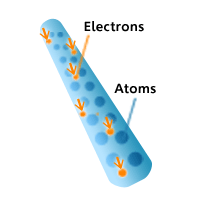# Potential difference and current

When discussing electrical energy there are several important quantities that must be understood.

## Potential Difference or Voltage (V)

This tells you how much energy is available to push electric charges through a circuit. To a physicist, it is a measure of the "Electrical potential energy difference that a charge has at one point compared to another."

A familiar way to understand potential difference is to think of a stone on the top of a hill. The stone has a lot of gravitational potential energy which it can lose if it is allowed to fall to the ground. In a battery, or power supply, the electric charges at one terminal (by convention the positive terminal) have MORE energy (electrical potential energy) than when they get to the other terminal, i.e. they can fall downhill from the positive terminal to the negative terminal. For the charges to lose their energy they require a conductive path to allow them to fall down the electrical hill. This path is an ELECTRIC CIRCUIT that provides a way for the charges to lose their excess energy as they travel around the circuit. This is just like the rock on the hill. If it is allowed to, it can fall to the "ground" and lose the stored energy it had.

The energy the charges have to lose is referred to as the 'potential difference'. The potential difference tells you how much energy per coulomb the charges have to lose. Potential difference is measured in volts, symbol V, where 1 volt is equivalent to an energy difference of 1 joule for each coulomb of charge. Potential difference is commonly referred to as voltage.

## Electric current (I)This is the rate at which the electric charges flow through the circuit, i.e. How quickly the electric charges are moving through the circuit. An ammeter is used in a circuit to record the current. Electric current has the symbol I and is measured in ampere (symbol A) where 1 ampere is equivalent to a flow of 1 coulomb per second. Although current was originally thought to be a flow of positive charge, we now know that in most cases it is only the very tiny negatively charged electrons that flow through wires as a current.

Remember the positive charge is carried by the proton and they are all held very tightly in the nucleus of an atom so unless the subtance is a fluid positive charge, it cannot move easily in a substance.

## Electric charge (Q or q)

This is what makes something electric. All atoms contain electrically charged components. The positive charge is produced by the protons that are held together with uncharged neutrons in the nucleus of atoms. The negative charge is produced by the electrons. The electrons are the smallest particles in an atom and orbit around the nucleus at relatively large distances (a bit like the tiny Earth orbiting the Sun).The charge of an electron is exactly the same as the charge of a proton. An electric charge is usually due to a deficiency or excess of electrons. Most substances are normally neutral with equal amounts of positive and negative charge.